Learning Library
Who we are
Guidance

# 5.NBT.A.3.A Worksheets, Workbooks, Lesson Plans, and Games

#### CCSS.MATH.CONTENT.5.NBT.A.3.A

:
"Read and write decimals to thousandths using base-ten numerals, number names, and expanded form, e.g., 347.392 = 3 × 100 + 4 × 10 + 7 × 1 + 3 × (1/10) + 9 × (1/100) + 2 × (1/1000)."

These worksheets, games, and lesson plans can help students practice this Common Core State Standards skill.

## Worksheets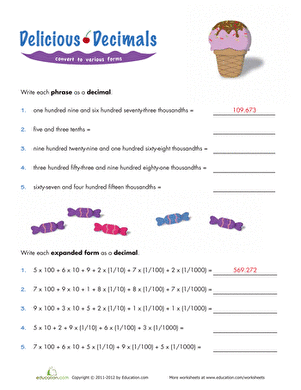Writing Decimals in Expanded Form
Worksheet
Writing Decimals in Expanded Form
This worksheet focuses on decimals and how to write them in expanded form using fractions.
Math
Worksheet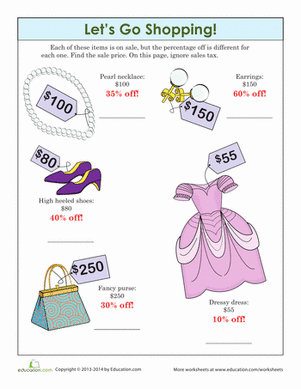Let's Go Shopping!
Worksheet
Let's Go Shopping!
Practice percentages by finding the discount prices on this quality merchandise.
Math
Worksheet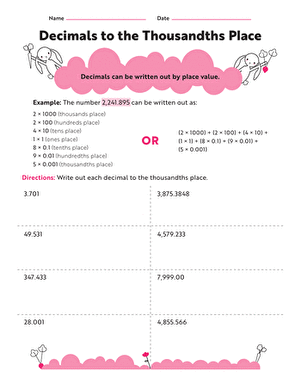Thousandth Decimal Place
Worksheet
Thousandth Decimal Place
Help out the perplexed rabbits on this worksheet and count your way to the thousandth decimal place in each problem.
Math
Worksheet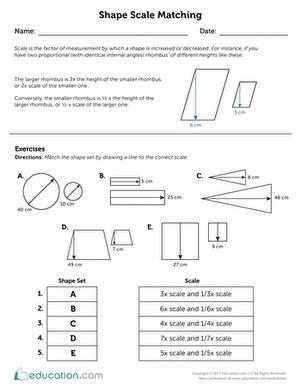Shape Scale Matching
Worksheet
Shape Scale Matching
Scale is the factor of measurement by which a shape is increased or decreased. Use this resource to teach your students about shapes to scale.
Math
Worksheet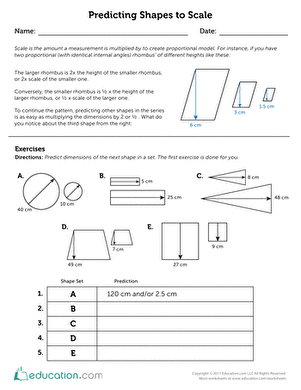Predicting Shapes to Scale
Worksheet
Predicting Shapes to Scale
What is scale? How do you scale shapes? Use this resource to help your students practice predicting scaled items in a series.
Math
Worksheet

## Lesson Plans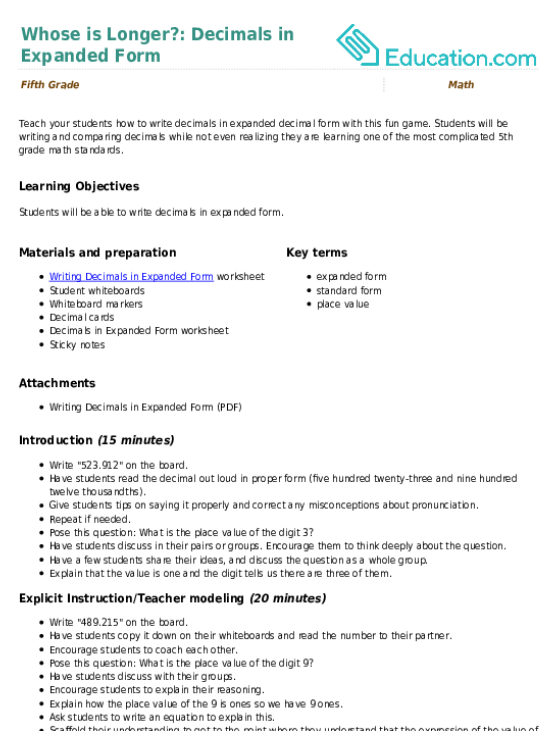Whose is Longer?: Decimals in Expanded Form
Lesson plan
Whose is Longer?: Decimals in Expanded Form
Teach your students how to write decimals in expanded decimal form with this fun game. Students will be writing and comparing decimals while not even realizing they are learning one of the most complicated 5th grade math standards.
Math
Lesson planBattle of the Tenths
Lesson plan
Battle of the Tenths
In this lesson, students will gain and use knowledge of expanded fraction form to play a fun card game involving rounding.
Math
Lesson plan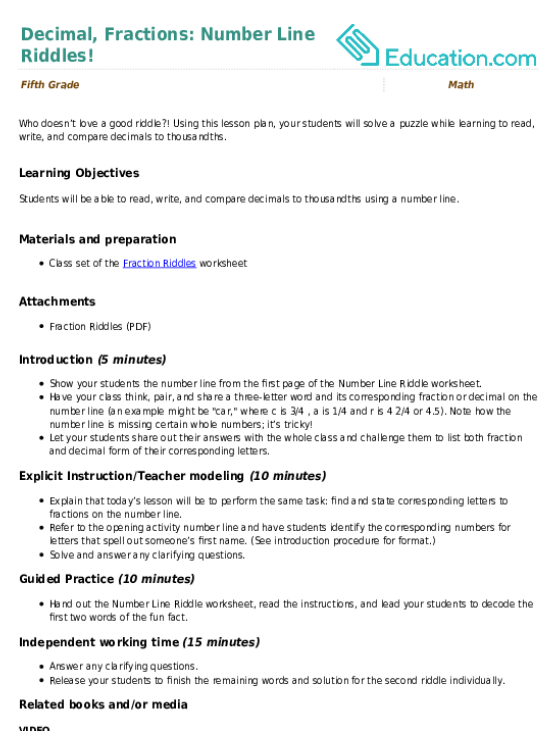Decimal, Fractions: Number Line Riddles!
Lesson plan
Decimal, Fractions: Number Line Riddles!
Who doesn’t love a good riddle?! Using this lesson plan, your students will solve a puzzle while learning to read, write, and compare decimals to thousandths.
Math
Lesson planScale, Shapes, and Sets
Lesson plan
Scale, Shapes, and Sets
Use this fun and easy lesson plan to teach your students to predict and calculate dimensions for shapes to scale in sets.
Math
Lesson plan

## Workbooks

No workbooks found for this common core node.

## Games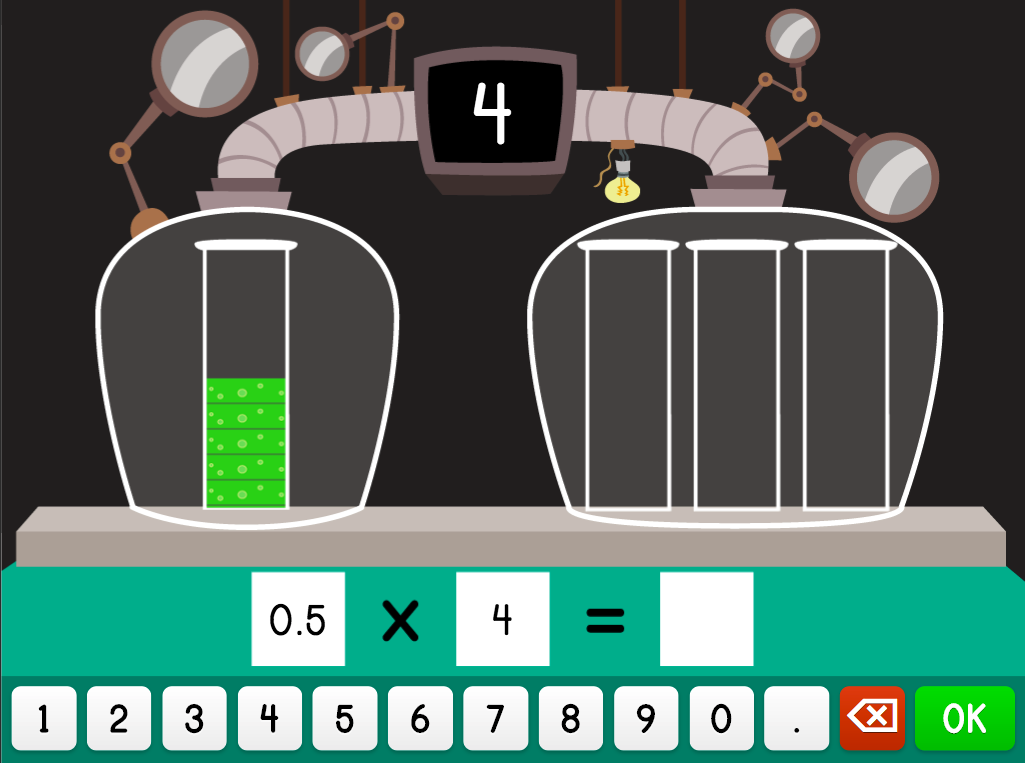Multiplying Decimal Numbers in Science
Game
Multiplying Decimal Numbers in Science
Kids learn how to multiply whole numbers and decimals in this super science game.